• n个小数待分组，每个数组k个，如果n%k==0,那么组数为n/k个，如果n%k!=0,则最后一组的数量为n%k,组数为n/K+1,要求每个组的均值相等或者相近！java
• 数据结构与算法 时间复杂度与空间复杂度 时间复杂度 并不是计算程序具体运行的时间，而是算法执行语句的次数。 O(2^n) 表示对n数据处理需要进行 2^n 次计算。 O(1), O(log(n)), O(n^a) 多项式时间复杂度 O(n^a) 和O...
说明
讲师：李智慧
数据结构与算法
时间复杂度与空间复杂度
时间复杂度
并不是计算程序具体运行的时间，而是算法执行语句的次数。O(2^n) 表示对n数据处理需要进行 2^n 次计算。O(1), O(log(n)), O(n^a) 多项式时间复杂度O(n^a) 和O(n!) 非多项式的时间复杂度。
空间复杂度
一个算法在运行过程中临时占用存储空间大小的量度。O(n) 表示需要临时存储n个数据。NP问题
P问题：能在多项式时间复杂度内解决的问题。NP问题：能在多项式时间复杂度内验证答案正确与否的问题。NP ?= PNP-hard 问题：比NP问题更难的问题（NP问题的解法可以规约到NP-hard问题的解法）NP完全问题：是一个NP-hard问题，也是一个NP问题。
NP问题举例：数独填写，横竖都是填写不重复的1~9个数， 方块也是写的1~9个数。访问全部地区的最省方式。数组
创建数组必须要内存中一块连续的空间。 数组中必须存放相同的数据类型。 随机快速读写是数组的一个重要特性，根据数组的下标访问数据，时间复杂度为 O(1).链表
链表可以使用零散的内存空间存储数据。 所以链表中的每个数据元素都必须包含一个指向下一个数据元素的内存地址指针。要想在链表中查找一个数据，只能遍历链表，所以链表的查询时间复杂度总是O(N).链表中增删数据要比数组性能好的多链表增删数据时间复杂度总是O(1).数组增删数据时间复杂度总是O(N).
数组链表结合，实现快速查找和快速增删Hash 表
如何既快速访问数据，有快速增删数据？Hash表查、增、删数据时间复杂度总是O(1).
Hash 冲突如果Hash(Key)的位置，存储不止一个值，就挨个匹配key值，取出相应的value值。
栈
数组和链表都被称为线性表。栈就是在线性表的基础上加了这样的操作限制条件：后面添加的数据，在删除的时候必须先删除，即通常所说的“后进先出”。
线程运行时专有内存为什么被称为线程栈？
线程调用栈
void f() {
int x = g(1);
x++;	// g 函数返回，当前堆栈顶部为f函数栈帧，在当前栈帧继续执行f函数的代码。
}

int g(int x) {
return x + 1;
}队列
队列也是一种受限的线性表，栈是后进先出，而队列是先进先出。
典型的应用场景：生产者消费者；阻塞等待的线程被放入队列。用队列搜索好友中关系最近的有钱人构建一个队列：
把你入队列。然后把你出队列，把你的好友Bob, Alice, Claire入队列。看看是否是有钱人？如果不是。把Bob，Alice，Claire依次出队列，把他们的好友入队列。
用队列搜索最短路径CAB先入队列，CAB出队列，把CAB的临近结点CAT，CAR入队列。CAT，CAR相继出队列，把它们的临近结点BAT入队列，发现这就是终点。也就是最短路径是CAB - CAT - BAT.
树二叉排序树
左子树上所有节点的值均小于或等于它的根节点的值。右子树上所有节点的值均大于或等于它的跟节点的值。左、右子树也分别为二叉排序树。时间复杂度：O(logn)
不平衡的二叉排序树不平衡的二叉排序树退化为链表，时间复杂度：O(n)
平衡二叉（排序）树
从任何一个结点出发，左右子树深度只差的绝对值不超过1. 左右子树仍然为平衡二叉树。旋转二叉树恢复平衡
插入式，最多只需要两次旋转就会重新恢复平衡。
删除时，需要维护从被删结点到根节点这条路径上所有节点的平衡性，时间复杂度为O(logn).右旋的过程：
15变成根节点；17比15大，则17是15的右节点。16比17小，则16是17的左节点。
左旋的过程：
25变成根节点；22比25小，则22是25的左节点。23比22大，则23是22的右节点。
红黑（排序）树
每个节点只有两种颜色：红色和黑色。根节点是黑色的。每个叶子节点(Null)都是黑色的空节点。从根节点到叶子节点，不会出现两个连续的红色节点。从任何一个节点出发，到叶子节点，这条路径上都有相同数目的黑色节点。增删结点的时候，红黑树通过染色和旋转，保持红黑树满足定义TreeMap就是用红黑树实现的。
红黑树 vs 平衡二叉树
红黑树最多只需3次旋转就会重新达成红黑平衡，时间复杂度O(1).在大量增删的情况下，红黑树的效率更高。红黑树的平衡性不如平衡二叉树，查找效率要差一些。
跳表时间复杂度：O(logn) 空间复杂度：O(n)
常用算法
穷举算法递归算法贪心算法动态规划
递归算法（快速排序算法）
def quicksort(array):
if	len(array) < 2:
return array	// 基线条件： 为空或只包含一个元素的数组是 “有序”的
else:
pivot = array	// 递归条件
less = [i for i in array[1:] if i <= pivot]	// 由所有小于基准值的元素组成的子数组
greater = [i for i in array[1:] if i > pivot]	// 由所有大于基准值的元素组成的子数组
return quicksort(less) + [pivot] + quicksort(greater)

print quicksort([10, 5, 2, 3])

注意： 递归算法一定要有退出条件，否则就会栈溢出 Stack Overflow. 栈的空间是有限的，所以函数调用不能太深，比如写个计数，超过1000就退出。
时间复杂度：O(n * log(n))
贪心算法
背包问题： 拿一个装4磅中背包到商城买东西，将哪些商品放入背包才能受益最大化。每一步都找当前的最优价，但是不一定是结果的最优解。
音响 = 4磅，3000美元。笔记本 + 吉他 = 4磅， 4500美元。
改进贪心算法 - 迪杰斯特拉算法（最快路径）迪杰斯特拉算法（最快路径）
找出 “最便宜” 的节点，即可在最短时间内到达的节点。更新该节点的邻居的开销，检查是否有前往它们的更短路径，如果有，就更新其开销。重复这个过程，直到对图中的每个节点都这样做了。计算最终路径。迪杰斯特拉算法的核心是找到起点到每个节点的最快路径。动态规划
动态规划算法解决背包问题： 通过找到合适的角度，将所求解的目标值在某（几）个维度上展开，将一个大问题拆解为若干小问题，小问题的最优解，寻找大问题的最优解。每个动态规划算法都是从一个网格开始，背包问题的网格如下：遗传算法不一定得到最优解
遗传算法过程遗传算法解决背包问题
遗传算法( Genetic Algorithm, GA ) 是模拟达尔文生物进化论的自然选择和遗传学机理的生物进化过程的计算模型，是一种通过模拟自然进化过程搜索最优解的方法。
遗传算法以一种群体中的所有个体对象，并利用随机化技术指导对一个被编码的参数空间进行高效搜索。其中，选择、交叉和变异构成了遗传算法的遗传操作；参数编码、初始群体的设定、适应度函数的设计、遗传操作设计、控制参数设定五个要素组成了遗传算法的核心内容。
例子：80公斤背包装哪些物品价值最大？
物品重量价值110152152532035425455305563570
基因编码与染色体
物品基因编码
物品1物品2物品3物品3物品5物品6染色体111111111111
基因组合：染色体 选择初始染色体：随机产生(也可以人为或者算法选择)
100100， 对应物品1、物品4存在。101010， 对应物品1、物品3、物品5存在。010101， 对应物品2、物品4、物品6存在。101011， 对应物品1、物品3、物品5、物品6存在。
适应函数与控制参数
选择适应度函数，这里为商品总价值。
100100 = 15 + 45 = 60.101010 = 15 + 35 + 55 = 105.010101 = 25 + 45 + 70 = 140.101011 = 15 + 35 + 55 + 70 = 175.
选择控制参数，这里为重量
100100 = 10 + 25 = 35.101010 = 10 + 20 + 30 = 60.010101 = 15 + 25 + 35 = 75.101011 = 10 + 20 + 30 + 35 = 95. 染色体101011超重，淘汰。
选择算法
在剩下的染色体中选择可以被遗传下去的染色体以及繁殖次数。
100100， 适应度函数为 60.101010， 适应度函数为 105.010101， 适应度函数为 140.
选择算法
轮盘选择 ( Roulette Wheel Selection ): 是一种回放式随机采样方法。每个染色体进入下一代的概率等于它的适应度值与整体适应度值和的比例。随机竞争选择( Stochastic Tournament): 每次按轮盘赌选择一对个体，然后让这两个个体进行竞争，适应度高的被选中，如此反复，直到选满为止。均匀排序：对群体中的所有个体按照适应度大小进行排序，基于这个排序来分配各个个体被选中的概率。
交叉遗传
选中结果： 101010 和 010101 个繁殖两次
生产下一代：染色体交叉遗传
个体染色体配对交叉点位置交叉结果11010101-2310110120101011-2301001031010103-4410100120101013-44010110
循环迭代，如果连续几代遗传都没有出现更强的染色体（价值总和更大），那么算法收敛，当前最大价值的染色体为最终解。
有时候会在遗传过程中进行基因突变，得到基因突变染色体。
时间复杂度：O(1)
展开全文• 此ppt介绍了解决TSP（旅行商问题）的三种算法：动态规划、蚁群算法、遗传算法
• 本案例实现了遗传算法好非线性规划函数的结合，可用于动态路径规划，也可用于工程实践，函数寻优。
• 研究针对遗传算法（GA）提出了一种新的变异算子，并将其应用于动态环境下移动机器人的路径规划问题。移动机器人的路径规划在障碍物环境中发现从起始节点到目标节点的可行路径。 通过利用其强大的优化能力，遗传算法...
• 考虑EV保有量增长的影响，同时计及EV增长率的不确定性，构建了2种EV充电站随机机会约束动态规划模型，并提出考虑充电需求空间分布的改进自适应遗传算法(IAGA)求解上述规划模型。通过一个实际算例验证了所提IAGA在...
• 经典算法问题-TSP商旅问题（Traveling Salesman Problem），它是数学领域中著名问题之一。...代码包含遗传算法动态规划来求解这个问题，里面有完整源代码，并且有详细注释，还有两者的比较分析。
• 动态规划,分治算法,概率算法,模拟退火算法,搜索算法,贪婪算法,网上matlab,遗传算法,组合算法.分治算法 概率算法 模拟退火算法 搜索算法
•研究论文
• 动态环境下，有几个大小不同的障碍物，指定起始点和终点，根据遗传算法规划出一条无碰撞的路径！
• 在此基础上运用遗传算法获得考虑负荷变化情况下配电网低压侧无功补偿装置的动态最优规划结果。详细讨论了投资规模不加限制、限制总投资规模和限制各个阶段投资规模三种情况的处理方法。对实例的分析表明。提出的方法...
• 基于单亲遗传算法混合动态规划的电动汽车充电调度优化策略.pdf
• 探讨了一种改进的基于遗传算法的静态环境下机器人全局路径规划方法的可行性。该方法通过障碍物的数量来动态确定所需的路径点数（基因），使得它能更广泛地应用于不同环境,最后对结果进行修正。仿真实验表明了该方法...移动机器人 适应度函数
• 为处理遗传算法迭代过程中产生的不可行解，引入了基于罚函数法的 约束处理方法。针对罚函数法中惩罚系数难以确定的特点，设计了惩罚系数自适应调整的动态罚函数机制。 根据模拟的数据进行实验及分析，表明该方法能...
• 动态环境中基于遗传算法的移动机器人路径规划的方法pdf,动态环境中基于遗传算法的移动机器人路径规划的方法
• 路径规划主要是让目标对象在规定范围内的区域内找到一条从起点到终点的无碰撞安全路径。路径规划中有静态路径规划以及动态路径规划，本文所讨论的...本文采用遗传算法和模拟退火混合遗传算法进行求解，得到最优路径。


文章目录
一、问题描述二、遗传算法设计2.1 算法原理2.2 编码2.2 适应度函数2.3 混合遗传算法
三、实验结果及分析四、总结参考文献MATLAB代码主程序相关函数

一、问题描述
路径规划主要是让目标对象在规定范围内的区域内找到一条从起点到终点的无碰撞安全路径。路径规划中有静态路径规划以及动态路径规划，本文所讨论的问题仅针对静态路径规划。具体问题描述如下： 给定起点、终点和障碍物等环境信息，如图1.1所示，利用演化计算方法得到最优轨迹，并分析不同参数选择对结果的影响。本文采用遗传算法和模拟退火混合遗传算法进行求解，得到最优路径。二、遗传算法设计
2.1 算法原理
遗传算法（Genetic Algorithm，GA）是进化计算的一部分，是模拟达尔文的遗传选择和自然淘汰的生物进化过程的计算模型，是一种通过模拟自然进化过程搜索最优解的方法。该算法简单、通用，鲁棒性强，适于并行处理。基本遗传算法流程包括种群初始化、选择、交叉、变异等操作，如图2.1所示。遗传算法的进化过程从完全随机生成的种群开始，随后逐代向适应度更高的种群进化。在进化的每一代中，整个种群的适应度按照一定规则被评价，基于个体的适应度高低从当前种群中随机选择多个个体，通过个体自然选择和基因突变操作而产生新种群，生成的新种群在算法的下一次迭代中成为当前种群。在遗传算法中，需解决的问题的解通常被成为基因个体，它通常以参数列表的形式进行标示，被称作染色体或基因串。其中染色体通常以编码形式体现，即表达为简单的字符串或数字串。
针对本问题，使用遗传算法求解最优路径需要按照特定规则初始化种群，并且根据问题所给的限定条件设计合适的适应度计算方法。下面的内容会详细说明这两个步骤，而算法中的其他操作，如选择、交叉、变异分别采用经典的轮盘赌、单点交叉和随机变异方法，这里不再赘述。
2.2 编码
针对两点间避障路径规划问题，考虑将种群中的每一个个体作为一条路径，个体由一系列坐标点

(

x

,

y

)

(x, y)

组成，所以个体的染色体长度为坐标点数。为了编程方便，这里将个体的坐标分开存储，得到两个种群

P

o

p

x

Popx

和

P

o

p

y

Popy

，在进化过程中将两个种群看作一个种群，共同优化。

P

o

p

x

i

=

(

x

1

,

x

2

,

…

,

x

j

)

,

P

o

p

y

i

=

(

y

1

,

y

2

,

…

,

y

j

)

.

Pop{x_i} = ({x_1},{x_2}, \ldots ,{x_j}),Pop{y_i} = ({y_1},{y_2}, \ldots ,{y_j}).

其中

P

o

p

x

i

Popx_i

和

P

o

p

y

i

Popy_i

表示种群中的第i个个体，

i

∈

[

1

,

M

]

i \in \left[ {1,M} \right]

；

x

j

x_j

和

y

j

y_j

为个体染色体中的一对编码，表示一个点的横纵坐标，

j

∈

[

1

,

N

]

j \in \left[ {1,N} \right]

；

M

M

和

N

N

分别表示种群数量和染色体长度。
2.2 适应度函数
适应度函数要有效反映每一个体与问题的最优个体之间的差距。遗传算法在进化搜索中基本不利用外部信息,仅以适应度函数为依据,利用种群中每个个体的适应度值来进行搜索。本问题的目标是路径总长度为最短，路径总长度的倒数就可以作为适应度函数：

F

i

t

n

e

s

s

=

1

∑

i

=

1

M

−

1

(

x

i

+

1

−

x

i

)

2

+

(

y

i

+

1

−

y

i

)

2

{\rm{ Fitness }} = \frac{1}{{\sum\limits_{i = 1}^{M - 1} {\sqrt {{{({x_{i + 1}} - {x_i})}^2} + {{({y_{i + 1}} - {y_i})}^2}} } }}

式中，

x

i

x_i

和

y

i

y_i

是种群中第i个个体的坐标；

M

M

为种群规模。
为了计算距离，需要判断点和两点连线与障碍物的关系，即判断个体染色体的

x

，

y

x，y

编码对是否在圆形障碍物内，以及相邻编码对所连成的线段是否与圆形障碍物有交点。若存在点

(

x

,

y

)

(x, y)

不满足上式的条件，则将该个体的适应度置零。

(

x

−

x

0

)

2

+

(

y

−

y

0

)

2

>

r

2

{(x - {x_0})^2} + {(y - {y_0})^2} > {r^2}

经过上一步的判断，符合条件的个体编码坐标点都在圆形障碍物外，所以这时判断相邻两坐标点组成的线段与障碍物的关系可分为如图2.2所示的三种情况讨论：在分情况讨论之前，还需要计算圆心到直线的距离

d

d

，这是为了区别圆心在直线上的特殊情况。计算公式为：

d

=

∣

A

x

0

+

B

y

0

+

C

∣

A

2

+

B

2

d = \frac{{\left| {A{x_0} + B{y_0} + C} \right|}}{{\sqrt {{A^2} + {B^2}} }}

对于第一种情况和第二种情况，

d

<

r

d<r

。计算

∠

O

A

B

\angle OAB

和

∠

O

B

A

\angle OBA

的余弦函数，若都大于0则该线段与圆相交，不符合条件，将该个体的适应度置零。计算公式为：

cos

⁡

∠

O

A

B

=

A

O

→

⋅

A

B

→

∣

A

O

→

∣

∣

A

B

→

∣

>

0

⇒

A

O

→

⋅

A

B

→

>

0

\cos \angle OAB = \frac{{\overrightarrow {AO} \cdot \overrightarrow {AB} }}{{\left| {\overrightarrow {AO} } \right|\left| {\overrightarrow {AB} } \right|}} > 0 \Rightarrow \overrightarrow {AO} \cdot \overrightarrow {AB} > 0

对于第三种情况，需要判断BA和BO的距离，

d

=

0

d=0

。若满足

∣

B

A

→

∣

>

∣

B

O

→

∣

\left| {\overrightarrow {BA} } \right| > \left| {\overrightarrow {BO} } \right|

，则证明该线段穿过圆，不符合条件，将该个体的适应度置零。
具体地，计算适应度函数的算法为：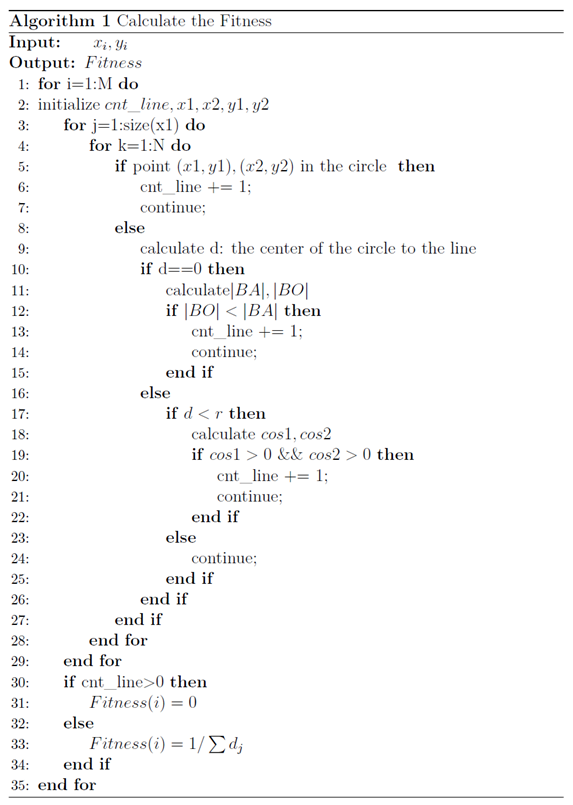2.3 混合遗传算法
为了提高遗传算法运行效率和求解质量，这里引入模拟退火算法的思想，模拟退火(Stimulated Annealing, SA)具有较强的局部寻优能力，并能使搜索过程避免陷入局部最优解[3-4]，采用模拟退火遗传算法（Stimulated Annealing Genetic Algorithm，SAGA）求解路径优化问题。
具体来说，模拟退火遗传算法是对适应度函数的改进，在前期（高温）减少个体间的适应度差异，后期（低温）放大个体间适应度差异，突出优秀个体，完成适应度拉伸的作用，在一定程度上弥补了遗传算法在前期容易早熟、后期容易停滞的缺点。具体的适应度值计算公式如式（6）和（7）所示：

f

i

=

e

f

i

T

∑

i

=

1

M

e

f

i

T

{f_i} = \frac{{{e^{\frac{{{f_i}}}{T}}}}}{{\sum\limits_{i = 1}^M {{e^{\frac{{{f_i}}}{T}}}} }}

T

=

T

0

(

A

g

−

1

)

T = {T_0}({A^{g - 1}})

其中，

M

M

为种群大小，

f

i

f_i

为第

i

i

个个体的适应度，

g

g

为遗传代数，

T

T

为温度，

T

0

T_0

为初始温度，

A

A

为退火速度（取0.99）。
三、实验结果及分析
本文实验环境为MATLAB2018b，探讨遗传算法的参数设置对结果的影响，比如迭代次数、交叉概率、变异概率，同时比较在相同参数条件下基本遗传算法和混合遗传算法的性能。最后通过可视化结果和路径距离长度来评估算法的准确性与有效性。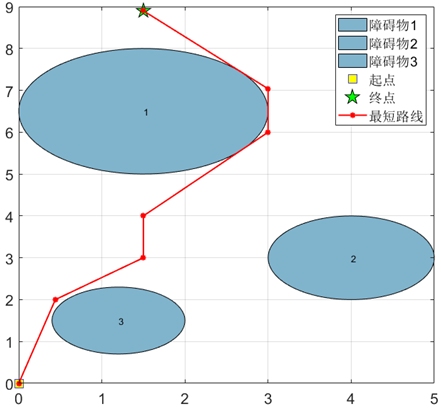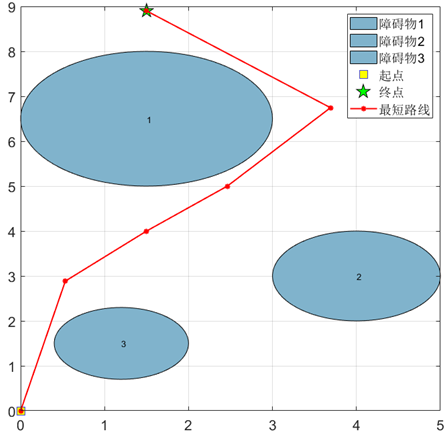四、总结
本次作业通过遗传算法编程求解了障碍物路径规划问题，对于基本遗传算法，探讨了算法中迭代次数、交叉概率和变异概率等参数对优化结果的影响。实验结果表明迭代次数不能设置得过小，否则算法还未收敛，反之则会导致计算资源的浪费；交叉概率和变异概率的大小影响种群的多样性，这两个参数过大会导致震荡，无法收敛，反之会导致优化出现停滞。此外，还将模拟退火的思想引入遗传算法，采用模拟退火遗传算法对本问题进行优化。实验结果证明GA算法更适用于该问题的优化求解，这是由于对该问题的个体坐标点排序和SAGA更强局部搜索能力导致无法使搜索过程进入最有希望的搜索区域共同造成的，同时SAGA较多的参数量也对算法寻优调参带来困难。
参考文献
 Holland J H. Genetic algorithms[J]. Scientific american, 1992, 267(1): 66-73.  王小平, 曹立明. 遗传算法——理论、应用与软件实现[M]. 西安交通大学出版社, 2002.  Kirkpatrick S, Gelatt C D, Vecchi M P. Optimization by simulated annealing[J]. science, 1983, 220(4598): 671-680.  王雪梅,王义和.模拟退火算法与遗传算法的结合[J].计算机学报,1997(04):381-384.  Sen M K, Datta-Gupta A, Stoffa P L, et al. Stochastic reservoir modeling using simulated annealing and genetic algorithm[J]. SPE Formation Evaluation, 1995, 10(01): 49-56.
MATLAB代码
主程序
GA_main.m
%%%%%%基本遗传算法(GA)%%%%%%%%%%%

clear
%%%设置超参数
p_crs = 0.7;   %交叉概率
p_mut = 0.1;   %变异概率
ratio = 0.5;   %选择操作中父辈的比例
pop_num = 5000;  %种群规模
chrom_len = 7;  %染色体长度，这里代表路线的点数
iteration = 40;

% 一个个体就是一条路线
[x,y]=popinit(pop_num,chrom_len);   %产生初始种群
fit=fitness(x,y);      %计算种群适应度
[bestx0,besty0,fit0]=best(x,y,fit);

for i=1:1:iteration           %设置进化代数
[Parentx,Parenty]=select(x, y, fit, ratio);      %选择
[Kidx,Kidy]=crossover(Parentx,Parenty,p_crs);       %交叉
[Kidx,Kidy]=mutation(Kidx,Kidy,p_mut);              %变异
x = [Parentx; Kidx];    % 得到新的种群
y = [Parentx; Kidy];
x(:,chrom_len)=1.5;  %保留终点
y(:,chrom_len)=8.9;

fit = fitness(x,y);   % 计算进化后的适应度
[bestx,besty,bestfit]=best(x,y,fit);   %选择每一代中的最佳个体
route_x(i,:)=bestx;                     %保存该最佳个体
route_y(i,:)=besty;
route_fit(i)=bestfit;
fprintf('%dth 代进化完成...\n', i)
%     plot(bestx,besty,'r-');
end
route_fit = [fit0, route_fit];     %加上初始种群中最优个体
route_x = [bestx0; route_x];
route_y = [besty0; route_y];

[final_fit,idx]=max(route_fit);            %所有代中的的最佳路线
final_routex=route_x(idx,:);
final_routey=route_y(idx,:);
final_distance = 1.0/final_fit             %最佳路径长度

%==========画图，可视化路线、进化过程==============
% start point
xs=0;
ys=0;
% Destination
xt=1.5;
yt=8.9;
%obstacle
xobs=[1.5 4.0 1.2];
yobs=[6.5 3.0 1.5];
robs=[1.5 1.0 0.8];
theta=linspace(0,2*pi,100);
max_area = 0;
for k=1:numel(xobs)
fill(xobs(k)+robs(k)*cos(theta),yobs(k)+robs(k)*sin(theta),[0.5 0.7 0.8]);  % 后一个参数表示RGB值
text(xobs(k), yobs(k), num2str(k))
hold on;
end
plot(xs,ys,'bs','MarkerSize',12,'MarkerFaceColor','y');
plot(xt,yt,'kp','MarkerSize',16,'MarkerFaceColor','g');
grid on;
hold on;

%%画出最短路径的路线
plot(final_routex,final_routey,'r*-',  'linewidth', 1.5);
legend('障碍物1','障碍物2','障碍物3','起点', '终点', '最短路线')
set(gca,'FontSize',16);
hold off;

% 进化过程中适应度曲线
figure,
plot(0:1:size(route_fit,2)-1, route_fit, 'linewidth', 1.2)
ylim([0.08,0.1])
title(['变异率=',num2str(p_mut),',交叉率=', num2str(p_crs), '的进化曲线']);
legend('适应度值', 'Location', 'southeast');
set(gca,'FontSize',16);

figure,
plot(1./route_fit, 'linewidth', 1.2)
title(['变异率=',num2str(p_mut),',交叉率=', num2str(p_crs), '的进化曲线']);
legend('最短路径长度值', 'Location', 'northeast');
set(gca,'FontSize',16);

% d_ga = 1./route_fit;
% save('d_ga');


SAGA_main.m
%%%%%%模拟退火遗传算法(SAGA)%%%%%%%%%%%

clear
%%%设置超参数
p_crs = 0.7;   %交叉概率
p_mut = 0.1;   %变异概率
ratio = 0.5;   %选择操作中父辈的比例
pop_num = 5000;  %种群规模
chrom_len = 7;  %染色体长度，这里代表路线的点数
iteration = 40;
T0 = 100;  %初始温度
A = 0.8;  %退火速度

% 一个个体就是一条路线
[x,y]=popinit(pop_num,chrom_len);   %产生初始种群
fit=saga_fitness(x,y, T0);      %计算种群适应度
[bestx0,besty0,fit0]=best(x,y,fit);
d0 = 0; %初始路径长度
for j=1:1:size(bestx0,2)-1
d0 = d0 + sqrt((bestx0(1,j+1)-bestx0(1,j)).^2 + ...
(besty0(1,j+1)-besty0(1,j)).^2);     %该个体（即路线）的路径长度
end

for i=1:1:iteration           %设置进化代数
[Parentx,Parenty]=select(x, y, fit, ratio);      %选择
[Kidx,Kidy]=crossover(Parentx,Parenty,p_crs);       %交叉
[Kidx,Kidy]=mutation(Kidx,Kidy,p_mut);              %变异
x = [Parentx; Kidx];    % 得到新的种群
y = [Parentx; Kidy];
x(:,chrom_len)=1.5;   % 保留终点
y(:,chrom_len)=8.9;

T = T0 * A^(i-1);  % 当前温度
fit = saga_fitness(x,y,T);   % 计算进化后的适应度
[bestx,besty,bestfit]=best(x,y,fit);   %选择每一代中的最佳个体
route_x(i,:)=bestx;                     %保存该最佳个体
route_y(i,:)=besty;
route_fit(i)=bestfit;
for j=1:1:size(bestx,2)-1
dd(j)=sqrt((bestx(1,j+1)-bestx(1,j)).^2 + ...
(besty(1,j+1)-besty(1,j)).^2);     %该个体（即路线）的路径长度
end
d(i) = sum(dd);   %有问题
fprintf('%dth 代进化完成...\n', i)
%     plot(bestx,besty,'r-');
end
route_fit = [fit0, route_fit];   %加上初始种群中最优个体
route_x = [bestx0; route_x];
route_y = [bestx0; route_y];
d = [d0, d];

[final_fit,idx]=max(route_fit);            %所有代中的的最佳路线
final_routex=route_x(idx,:);
final_routey=route_y(idx,:);
final_distance = min(d)             %最佳路径长度

%==========画图，可视化路线、进化过程==============
% start point
xs=0;
ys=0;
% Destination
xt=1.5;
yt=8.9;
%obstacle
xobs=[1.5 4.0 1.2];
yobs=[6.5 3.0 1.5];
robs=[1.5 1.0 0.8];
theta=linspace(0,2*pi,100);
max_area = 0;
for k=1:numel(xobs)
fill(xobs(k)+robs(k)*cos(theta),yobs(k)+robs(k)*sin(theta),[0.5 0.7 0.8]);  % 后一个参数表示RGB值
text(xobs(k), yobs(k), num2str(k))
hold on;
end
plot(xs,ys,'bs','MarkerSize',12,'MarkerFaceColor','y');
plot(xt,yt,'kp','MarkerSize',16,'MarkerFaceColor','g');
grid on;
hold on;

%%画出最短路径的路线
plot(final_routex,final_routey,'r*-',  'linewidth', 1.5);
legend('障碍物1','障碍物2','障碍物3','起点', '终点', '最短路线')
set(gca,'FontSize',16);
hold off;

% 进化过程中适应度曲线
figure,
% plot(0:1:size(route_fit,2)-1, route_fit, 'linewidth', 1.2)
plot(d, 'linewidth', 1.2)
% ylim([0.08,0.1])
title(['变异率=',num2str(p_mut),',交叉率=', num2str(p_crs), '的进化曲线']);
legend('最短路径长度值', 'Location', 'northeast');
set(gca,'FontSize',16);

d_saga = d;
save('d_saga');

figure,
plot(d, 'linewidth', 1.2), hold on,
plot(d_ga, 'linewidth', 1.2);
title(['变异率=',num2str(p_mut),',交叉率=', num2str(p_crs), '的进化曲线']);
legend('SAGA最优路径值', 'GA最优路径值', 'Location', 'northeast');
set(gca,'FontSize',16);


相关函数
best.m
%====================================
%%输入参数：种群、其适应度
%%输出参数：种群中的最佳个体，及其适应度
%%说明：
%     选择最佳个体，便于后续分析
%====================================
function [bestx,besty,bestfit]=best(x,y,fitval)
bestx = x(1,:);
besty = y(1,:);
bestfit = fitval(1);
for i = 2:size(x,1)
if fitval(i) > bestfit
bestx = x(i,:);
besty = y(i,:);
bestfit=fitval(i);
end
end
end

crossover.m
%====================================
%%输入参数：父代种群，交叉概率
%%输出参数：子代种群
%%说明：
%     单点交叉，最后需要从小到大排序
%====================================
function [kidx, kidy]=crossover(x, y, p)
[m,n] = size(x);
kidx = ones(size(x));
kidy = ones(size(y));
for i = 1:2:m-1
if(rand < p)
point = round(rand*n);
kidx(i,:) = [x(i,1:point),x(i+1,point+1:n)];
kidx(i+1,:) = [x(i+1,1:point),x(i,point+1:n)];
kidy(i,:) = [y(i,1:point),y(i+1,point+1:n)];
kidy(i+1,:) = [y(i+1,1:point),y(i,point+1:n)];
else
kidx(i,:) = x(i,:);
kidx(i+1,:) = x(i+1,:);
kidy(i,:) = y(i,:);
kidy(i+1,:) = y(i+1,:);
end
end
for i = 1:1:m
kidx(i,:) = sort(kidx(i,:));
kidy(i,:) = sort(kidy(i,:));
end
end

fitness.m
%====================================
%%输入参数：种群——x,y横纵坐标
%%输出参数：种群中每个个体的适应度值
%%说明：
%     逐个计算种群中个体的适应度值，判断该个体所连成线路与圆形障碍物之间的关系，
%     将满足条件的路径的距离倒数作为适应度值
%====================================

function fitval=fitness(x,y)
%obstacle
xobs=[1.5 4.0 1.2];
yobs=[6.5 3.0 1.5];
robs=[1.5 1.0 0.8];
[n, xn] = size(x);
for i=1:1:n
cnt_line = 0;  %记录穿过障碍物的线段数

%%拆分相邻的两个点，便于后续计算
for m=1:1:xn-1
x1(m)=x(i,m);
y1(m)=y(i,m);
end
for n=2:1:xn
x2(n-1)=x(i,n);
y2(n-1)=y(i,n);
end

%%判断线段与障碍物的关系
for m = 1:size(x1,2)
A = y2(m) - y1(m);
B = x1(m) - x2(m);
C = y1(m)*(x2(m)-x1(m)) - x1(m)*(y2(m)-y1(m));
for ii = 1:3
if ((x1(m)-xobs(ii))^2 + (y1(m)-yobs(ii))^2) < robs(ii)^2 || ((x2(m)-xobs(ii))^2 + (y2(m)-yobs(ii))^2) < robs(ii)^2
% disp('有点在圆内')
cnt_line = cnt_line + 1;
continue;
else
d = abs(A*xobs(ii)+B*yobs(ii)+C) / sqrt(A^2+B^2);
if d==0
d1 = (xobs(ii)-x1(m))^2 + (yobs(ii)-y1(m))^2;
d2 = (x2(m)-x1(m))^2 + (y2(m)-y1(m))^2;
if d1 < d2
cnt_line = cnt_line + 1;   % 该线段过圆心且穿过圆
end

elseif d < robs(ii)
cos1 = (x2(m)-x1(m))*(xobs(ii)-x1(m)) + (y2(m)-y1(m))*(yobs(ii)-y1(m));
cos2 = (x1(m)-x2(m))*(xobs(ii)-x2(m)) + (y1(m)-y2(m))*(yobs(ii)-y2(m));
if cos1>0 && cos2>0
cnt_line = cnt_line + 1;    % 该线段与圆交与两点
end
else
continue;
end
end
end

end

%%%计算适应度
if cnt_line > 0
f(i)=0;       %路线穿过障碍物的个体适应度置零
else
for j=1:1:xn-1
d(j)=sqrt((x(i,j+1)-x(i,j)).^2+(y(i,j+1)-y(i,j)).^2);     %该个体（即路线）的路径长度
end
f(i)=1.0/sum(d);       %取距离的倒数作为个体适应度
end
record_cnt(i,:) = cnt_line;
end
fitval=f';
%     disp(['不满足条件的最小线段数为：',num2str(min(record_cnt, [], 1))])

end


mutation.m
%====================================
%%输入参数：父代种群，变异概率
%%输出参数：子代种群
%%说明：
%     随机替换，最后需要从小到大排序
%====================================
function [kidx, kidy]=mutation(x, y, p)
[m, n] = size(x);
kidx = x;
kidy = y;
for i = 1:1:m
if(rand < p)
point = round(rand*n);
% 避免越界
if point <= 1
point = 2;
end
if point == n
point = n-1;
end
% 变异：随机数替换
kidx(i,point) = round(rand*n);
kidy(i,point) = round(rand*n);
end
end
for i = 1:1:m
kidx(i,:) = sort(kidx(i,:));
kidy(i,:) = sort(kidy(i,:));
end
end

popint.m
%====================================
%%输入参数：种群数量，染色体长度
%%输出参数：初始种群
%%说明：
%     种群中的个体由一系列点组成，该点的x，y坐标分开存放，
%     除了起点和终点，其余点随机生成并从小到大排序
%====================================
function [x,y]=popinit(popsize,chromlength)

x=5.0*rand(popsize,chromlength);
y=8.9*rand(popsize,chromlength);
x(:,1)=0;  % 设置起点
y(:,1)=0;

for i=1:1:size(x,1)
x(i,:)=sort(x(i,:));
y(i,:)=sort(y(i,:));
end

x(:,chromlength)=1.5;  %设置终点
y(:,chromlength)=8.9;

end

saga_fitness.m
%====================================
%%输入参数：种群——x,y横纵坐标, 当前温度T
%%输出参数：种群中每个个体的适应度值
%%说明：
%     逐个计算种群中个体的适应度值，判断该个体所连成线路与圆形障碍物之间的关系，
%     将满足条件的路径的距离倒数作为适应度值
%====================================

function fitval=saga_fitness(x,y,T)
%obstacle
xobs=[1.5 4.0 1.2];
yobs=[6.5 3.0 1.5];
robs=[1.5 1.0 0.8];
[n, xn] = size(x);
for i=1:1:n
cnt_line = 0;  %记录穿过障碍物的线段数

%%拆分相邻的两个点，便于后续计算
for m=1:1:xn-1
x1(m)=x(i,m);
y1(m)=y(i,m);
end
for n=2:1:xn
x2(n-1)=x(i,n);
y2(n-1)=y(i,n);
end

%%判断线段与障碍物的关系
for m = 1:size(x1,2)
A = y2(m) - y1(m);
B = x1(m) - x2(m);
C = y1(m)*(x2(m)-x1(m)) - x1(m)*(y2(m)-y1(m));
for ii = 1:3
if ((x1(m)-xobs(ii))^2 + (y1(m)-yobs(ii))^2) < robs(ii)^2 || ((x2(m)-xobs(ii))^2 + (y2(m)-yobs(ii))^2) < robs(ii)^2
% disp('有点在圆内')
cnt_line = cnt_line + 1;
continue;
else
d = abs(A*xobs(ii)+B*yobs(ii)+C) / sqrt(A^2+B^2);
if d==0
d1 = (xobs(ii)-x1(m))^2 + (yobs(ii)-y1(m))^2;
d2 = (x2(m)-x1(m))^2 + (y2(m)-y1(m))^2;
if d1 < d2
cnt_line = cnt_line + 1;   % 该线段过圆心且穿过圆
end

elseif d < robs(ii)
cos1 = (x2(m)-x1(m))*(xobs(ii)-x1(m)) + (y2(m)-y1(m))*(yobs(ii)-y1(m));
cos2 = (x1(m)-x2(m))*(xobs(ii)-x2(m)) + (y1(m)-y2(m))*(yobs(ii)-y2(m));
if cos1>0 && cos2>0
cnt_line = cnt_line + 1;    % 该线段与圆交与两点
end
else
continue;
end
end
end

end

%%%计算适应度
if cnt_line > 0
f(i)=0;       %路线穿过障碍物的个体适应度置零
else
for j=1:1:xn-1
d(j)=sqrt((x(i,j+1)-x(i,j)).^2+(y(i,j+1)-y(i,j)).^2);     %该个体（即路线）的路径长度
end
f(i)=1.0/sum(d);       %取距离的倒数作为个体适应度
end
record_cnt(i,:) = cnt_line;
end

I = find(f ~= 0);
sumf = sum(exp(f(I) ./ T)) + n - size(I, 1);
f(I) = exp(f(I)./T) ./ sumf;
fitval=f';
%     disp(['不满足条件的最小线段数为：',num2str(min(record_cnt, [], 1))])

end

select.m
%====================================
%%输入参数：种群、其适应度、选择比例
%%输出参数：父辈种群——x,y横纵坐标
%%说明：
%     按照输入参数的比例，从种群中选择父代
%====================================
function [parentPopx, parentPopy]=select(popx, popy, fitness, ratio)
totalfit=sum(fitness); %适应值之和
accP=cumsum(fitness/totalfit); %概率累计和

%轮盘赌选择算法
for n=1:round(ratio*size(popx,1))
mat=find(accP>rand); %找到比随机数大的累积概率
if isempty(mat)
continue
end

parentPopx(n,:)=popx(mat(1),:);%将首个比随机数大的累积概率的位置的个体遗传下去
parentPopy(n,:)=popy(mat(1),:);
end
end


展开全文模拟退火 matlab 计算智能大作业
• 为此,文中 提出一种基于遗传算法的方法,其能够在合法解空间中快速收敛到最优解,满足动态规划 和管理的实时性要求。文中还提出了基于不同RAT频谱利用率差异的优化业务分布规划 方法,以进一步提升系统的整体资源利用率...
• 之前在网上找基于遗传算法的移动机器人路径规划资料的时候，没有找到理想的开源代码。最后参照论文，用matlab写了代码。平时学习的时候，会看很多人写的一些博客和公众号文章，觉得很有帮助，所以也想分享一点自己学...
  之前在网上找基于遗传算法的移动机器人路径规划资料的时候，没有找到理想的开源代码。最后参照论文，用matlab写了代码。最近开了公众号——Joe学习笔记，会不定期更新一些文章，主要是自己平时学到的知识，内容包括自动驾驶、计算机视觉、人工智能和机器人技术。我会第一时间把文章更新在公众号上，感兴趣的可以扫描下面的二维码关注并分享哦！最近新出了一个关于机器人路径规划和轨迹优化的视频课程，介绍常用的路径规划算法和轨迹优化算法，感兴趣的可以点击b站链接观看。更新中。。。 https://www.bilibili.com/video/BV1yT4y1T7Eb 　　遗传算法视频讲解地址：https://www.bilibili.com/video/BV1Sf4y1475E/
下面具体来讲讲基于遗传算法的移动机器人路径规划，文章是从公众号上搬过来的。 1.地图的建立和种群编码 　　机器人进行路径规划之前首先要建立地图，本文采用栅格法建立移动机器人的行走空间模型。栅格面积越小，空间中各种环境信息表示越精确，但同时占用的存储空间就会加大，算法所使用的搜索时间就会加大。若栅格面积越大，则空间中各种环境信息不能准确表示出来，容易出现碰撞问题。所以在建立环境模型时，需要先做以下规定： （1）不考虑障碍物高度问题，机器人行走空间为二维平面空间； （2）障碍物的大小、位置已知，并且不存在动态障碍物； （3）在规划时可以把机器人看做质点处理。 　　为了方便设置栅格面积，我们通常用正方形表示机器人的行走空间。如果障碍物面积小于正方形面积，可以将障碍物扩大为正方形；如果障碍物面积大于正方形面积，可以用两个正方形表示障碍物面积；如果障碍物面积更大，则可以用多个正方形表示。建模后机器人行走空间如图1所示，图中白色部分为自由栅格，黑色部分为障碍物栅格。在构建栅格地图时，首先以地图左下角第一个栅格为坐标原点建立直角坐标系。因此每一个栅格可以用（x,y）的坐标形式表示，比如左下角第一个栅格可以表示为（1,1）。并且从左下角开始每一个栅格进行编号N，编号从0开始。编号和坐标的转换公式为：Gsize为每一行栅格数，int为取整操作。已为每一个栅格编好号码，因此可以用此编号来表示一条路径，比如起点为0终点为24的路径可以表示为（0，6，7，8，13，19，24），在遗传算法中即为十进制编码。 2.代码整体框架 　　遗传算法是一种智能优化算法，主要用来解决优化问题，其主要步骤为种群初始化、适应度函数计算、选择、交叉和变异。应用于移动机器人路径规划时其主要步骤相同，流程图如图2所示。具体每步的方法将在以下小节做详细介绍。3.初始化种群 　　机器人的起始位置为栅格0，目标位置为栅格99。初始化种群要求随机产生多条可行路径，可行路径表示不与障碍物栅格相碰撞的路径。可行路径的产生分为两个主要步骤。第一步首先产生一条间断路径。机器人每次行走一个栅格，因此每一行至少有一个栅格在可行路径中。所以初始化时先按顺序在每一行随机取出一个无障碍栅格，形成一条间断的路径，其中为了减短路径长度路径的第一个和最后一个栅格分别为机器人的起始位置和目标位置。 　　第二步是将间断的路径连接为连续路径。在这一步中首先从第一个栅格开始判断相邻的两个栅格是否为连续栅格，栅格是否连续的判断方法为：若D等于1则说明两个相邻栅格连续，反之不连续。对于不连续的栅格取两个栅格的中点栅格，中点栅格的坐标计算为：若新栅格为障碍物栅格则以上下左右顺序取新栅格的相邻栅格，并判断此栅格是否已经在路径中（防止陷入死循环），如果此栅格为无障碍栅格且不在路径中则插入路径中，如果遍历上下左右四个栅格后没有满足条件的栅格则删除这条路径；若新栅格为无障碍物栅格，则插入两个不连续栅格中间。继续判断新插入的栅格和新插入的栅格的前一个栅格是否连续，若不连续则循环以上步骤，直到两个栅格连续。当两个栅格连续后取下一个栅格，循环以上步骤，直到整条路径连续。初始化一条路径的流程图如图3所示。很久没画框图了把框图的开始和结束画成了长方形，懒得改了将就看下吧。4.适应度函数计算 　　适应度函数分成两部分，分别用来判断路径的长短和平滑程度。路径长度的计算相对简单，公式如下：要求的是机器人的路径最短，而选择方式采用的是轮盘赌的方式，所以适应度函数的第一部分为：机器人由于运动学和动力学的约束，行进时拐弯不宜过大，并且相对平滑的路径有利于机器人的行驶，因此产生的路径有平滑度的要求。路径越平滑，相邻三点形成的角度越大，角度越大相邻三点之间的距离越大，因此计算路径中所有相邻三点的距离作为适应度函数的第二部分，计算公式如下：d3的值可对应路径中连续三点的夹角的四种情况分别为180度、钝角、直角和锐角，其中180度的三点成一直线，平滑度最好，钝角、直角次之。由于机器人动力学的约束，锐角是不允许的。所以分别对除直线外的3种情况给予惩罚，分别为5、30和无穷大，可以根据实际情况改动惩罚的大小。惩罚之和的倒数即为适应度函数的第二部分。 适应度函数的两部分需要取一个权重，权重公式如下：根据路径长度和平滑度之间的权重选择参数a和b。 5.选择方法 　　选择方法采用简单的基于概率的轮盘赌方法。首先计算出所有个体的适应度函数的和，再计算每一个个体所占的比率，计算公式如下：然后根据每个个体的概率，以轮盘赌的方式选择出下一代个体。轮盘赌的方式保证了部分非最优的个体，可以有效的防止算法陷入局部最优解。 6.交叉方式 　　首先需要确定一个交叉概率pc，产生0-1之间的一个随机数，并和交叉概率pc比较，若小于pc则进行交叉操作。交叉方式采用的是单点交叉的方式，具体的交叉操作是找出两条路径中所有相同的点，然后随机选择其中的一个点，将之后的路径进行交叉操作。具体的流程图如图4（a）所示，其中n为路径数（即种群数量）。 7.变异方式 　　首先需要确定一个变异概率pm，产生0-1之间的一个随机数，并和变异概率pm比较，若小于pm则进行变异操作。变异方法是随机选取路径中除起点和终点以外的两个栅格，去除这两个栅格之间的路径，然后以这两个栅格为相邻点，使用初始化路径中的第二步将这两个点进行连续操作。此时有可能无法产生连续的路径，则需要重新选择两个栅格执行以上操作，直到完成变异操作。具体的流程图如图3（b）所示，其中n为路径数（即种群数量）。（还是一样把开始和结束框画错了。）8.仿真结果 　　在20*20的栅格地图上进行实验，起点为0栅格，终点为399栅格，其中pc=0.8，pm=0.2，a=1，b=0。当b=0即表示不考虑路径的平滑度，只考虑了路径长度作为适应度函数，栅格地图上的路径如图5（a）所示，最短路径如图5（b）所示。从图5（a）中可以看到算法成功的找到了一条无障碍路径，但路径中有多处直角的转折，不利于机器人行驶，有时甚至出现锐角的情况，这种情况一般的机器人是无法行驶的。从图5（b）中得出，经过10次左右迭代算法已经收敛，最优路径的长度为31.799（相邻栅格间隔为1个单位长度）。当a=5，b=2时，即考虑路径平滑度时的算法结果如图5（c）、（d）所示，由于路径顺滑函数中对出现直角的情况加了较大的惩罚，出现锐角的情况加一个无穷大的惩罚，因此顺滑了路径且避免了锐角的出现。此时最优路径的长度也为31.799。9.总结 　　本文的重点是如何实现基于遗传算法的路径规划算法，首先介绍栅格地图的构建和十进制种群的编码方法。然后给出了整个过程的流程图，在此基础上详细介绍了种群初始化、适应度函数、选择方法、变异方法和交叉方法，并对其中较复杂的种群初始化、变异方法和交叉方法给出了程序流程图。主要的缺点是生成的路径不够平滑，可能是间断路径连续化产生的路径不够平滑，可以在此方面改进，也可以改进适应度函数中的路径平滑的部分，设计更加合理的平滑函数。 　　由于自己水平有限，其中的错误在所难免，大家发现错误可以在下面留言，不懂的地方也可以互相讨论。本文部分内容参考了文献：张小兵. 基于遗传算法的移动机器人路径规划[D]. 西安建筑科技大学, 2014.
展开全文• 动态环境中，移动机器人的动态路径规划是一个较难解决的课题．...同时，该方法充分挖掘了可应用遗传算法解决移动机器人动态路径规划的潜力．计算机仿真表明，仿真该控制方法具有良好的动态路径规划能力．
• 建立了无人作战飞机任务规划问题的数学模型, 提出了分层递阶的任务规划...心资源调度问题, 设计了基于遗传算法动态资源调度算法, 有效地解决了多无人作战飞机的资源调度问题. 计算结 果表明了算法的有效性.</p>
• 浅析遗传算法规划中应用前言一、遗传算法的原理(1)(1)(1)概念阐述自然选择遗传和变异(2)(2)(2)算法流程1.初始种群2.优胜劣汰3.遗传变异二、使用步骤1.引入库2.读入数据总结 前言 遗传算法（Genetic Algorithms，...


浅析遗传算法在规划中应用
前言一、遗传算法的原理

(

1

)

(1)

概念阐述自然选择遗传和变异

(

2

)

(2)

算法流程1.初始种群2.优胜劣汰3.遗传变异4.多目标优化问题中的非支配排序
二、路径问题的单目标遗传算法

前言
遗传算法（Genetic Algorithms，简称 GA）是一种基于自然选择原理和自然遗传机制的搜索（寻 优）算法，它是模拟自然界中的生命进化机制，在人工系统中实现特定目标的优化。遗传算法 的实质是通过群体搜索技术，根据适者生存的原则逐代进化，最终得到最优解或准最优解。 它必须做以下操作： • 初始群体的产生 • 根据适者生存的原则选择优良个体 • 被选出的优良个体两两配对 • 通过随机交叉其染色体的基因并随机变异某些染色体的基因后生成下一代群体 按照此方法使群体逐代进化， 直到满足进化终止条件。

一、遗传算法的原理

(

1

)

(1)

概念阐述
自然选择
自然选择（Natural selection ）指生物在生存斗争中适者生存、不适者被淘汰的现象，最初由C·R·达尔文提出。达尔文从生物与环境相互作用的观点出发，认为生物的变异、遗传和自然选择作用能导致生物的适应性改变。自然选择由于有充分的科学事实作根据，所以能经受住时间的考验，百余年来在学术界产生了深远的影响。 受自然界这种普遍机制的启发，我们可以将这种适者生存、不适者被淘汰用于我们数学中的

规

划

邻

域

\color{#00F}{规划邻域}

。给定一个系统的解

x

1

,

x

2

,

⋯

,

x

n

x_1,x_2,\cdots,x_n

,该解集中适者生存、不适者被淘汰，简单来说，就是对于系统规划较优的解能够保留下来，而其余解将被剔出。
遗传和变异
从生物学的角度来说，生命之所以能够一代一代地延续的原因，主要是由于遗传物质在生物进程之中得以代代相承，从而使后代具有与前代相近的性状。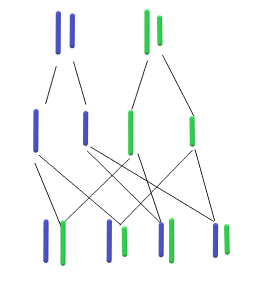如左图所示，母本和父本的染色体分离（其上所携带的基因也随之分离），在子代中，染色体上的基因两两组合生成下一代。 这里，我们可以假设染色体上的基因编码方式为二进制（

10010111010

⋯

\color{#F00}{10010111010\cdots}

）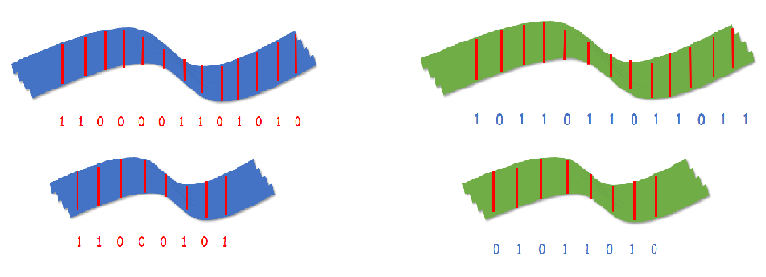交配之后，形成子代

1100001101010

1011011011011

\color{#F00}{1100001101010}\color{#00F}{1011011011011}

、

11000101

01011010

\color{#F00}{11000101}\color{#00F}{01011010}

，以上过程在数学上称为交叉。
变异，就是在染色体上的某一个位点，突然出现了一个新基因，代替了原有基因，这个基因叫做突变基因。于是后代的表现中也就突然地出现祖先从未有的新性状。采用二进制编码之后，变异如下图所示：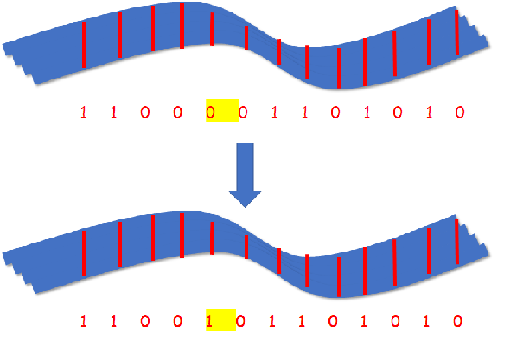一般来说，一个种群的变异的概率很小，变异具有不确定性。

(

2

)

(2)

算法流程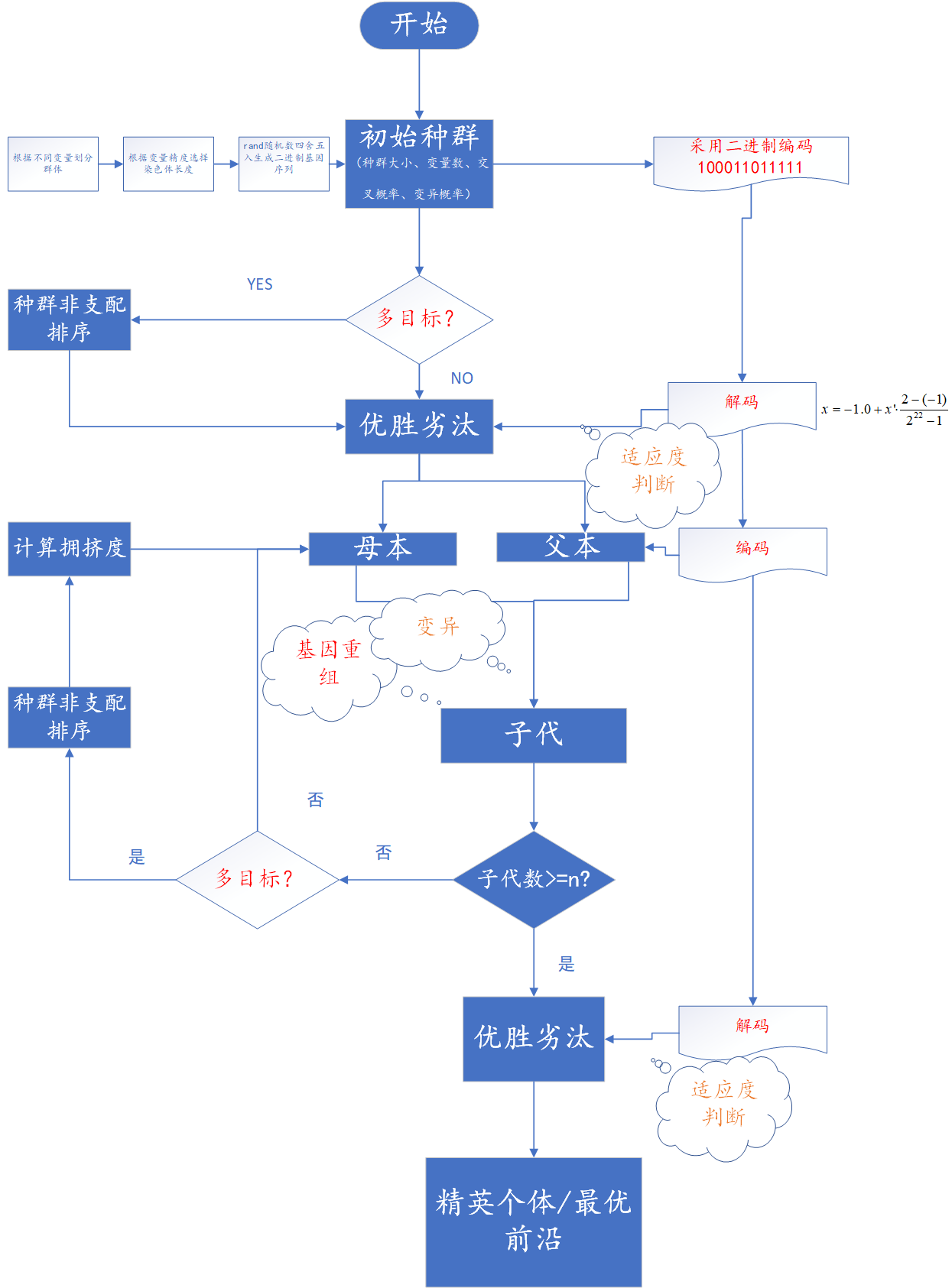1.初始种群
对于自然界来说，一个种群的繁衍必然依托于一个初始种群的诞生。没有初始种群，就没有后代，也就不会繁衍生息。初始种群是具有一定数目、一定规模无优良差异的特征群体。在算法中，初始种群对应一系列初值，好比一个函数的初值，一个项目的初始方案，一个路径规划中的初始路径

⋯

⋯

\cdots\cdots

这些所谓的初条件、初方案并没有优劣之分，于是算法流程中常常采用生成随机数（为了便于遗传和变异，常常采用随机生成二进制数列）来模拟这种

初

始

种

群

c

e

l

l

\color{#F00}{初始种群cell}

（

m

×

n

×

s

)

（m\times n \times s)

。
种群大小

m

m

随机二进制数列的行数种群大小越大，求解准确度提高物种个数

n

n

随机二进制数列的

胞

数

\color{#F00}{胞数}

=变量数适用于多元函数规划问题染色体长度

s

s

随机二进制数列的串长串长越长，精度越高（小数点后位数多）
例如：对于非线性规划问题

m

i

n

(

x

1

2

+

x

2

)

min ( x_1^2+x_2)

b

.

t

.

x

1

∈

[

−

1

,

2

]

b.t. x_1\in[-1,2]

x

2

∈

[

−

3

,

1

]

x_2\in[-3,1]

我们假设初始种群

m

=

5

m=5

由于变量为

x

1

,

x

2

x_1,x_2

两个，于是物种个数

n

=

2

n=2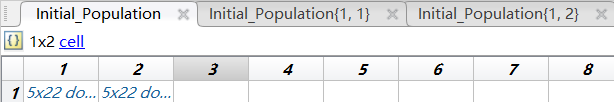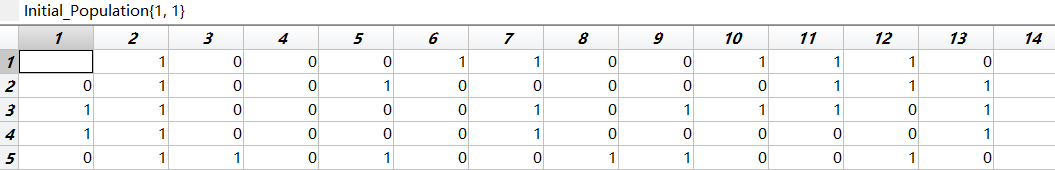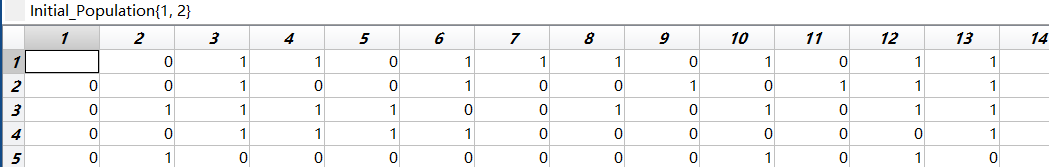2.优胜劣汰
首先介绍几个概念：

(

1

)

(1)

解码：将初始种群中各物种的二进制染色体翻译成十进制

x

′

x'

，

并

使

其

十

进

制

区

间

等

价

于

定

义

域

区

间

的

过

程

，

x

=

x

m

i

n

+

x

′

×

x

m

a

x

−

x

m

i

n

2

s

−

1

\color{#00F}{并使其十进制区间等价于定义域区间的过程}，x=x_{min}+x'\times \frac{x_{max}-x_{min}}{2^s-1}

。

b

.

t

.

x

1

∈

[

−

1

,

2

]

b.t. x_1\in[-1,2]

x

2

∈

[

−

3

,

1

]

x_2\in[-3,1]

变量

x

1

x_1

所产生的物种1号：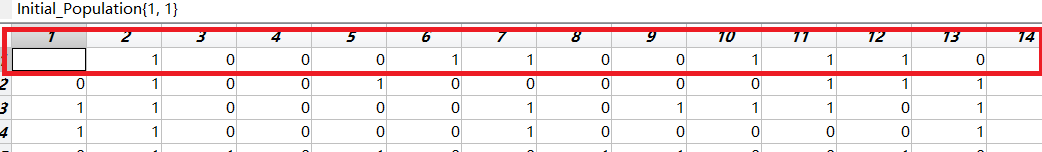编码为：0 1 0 0 0 1 1 0 0 1 1 1 0 0 0 1 0 1 0 0 0 0 化成十进制：

x

1

′

=

1154128

x_1'=1154128

编码结果:

x

1

=

−

1

+

1154128

×

2

−

(

−

1

)

2

22

−

1

=

−

0.174503

x_1=-1+1154128\times \frac{2-(-1)}{2^{22}-1}=-0.174503

(

2

)

(2)

适应度：种群中任意个体满足目标的程度。同样举个例子：还是这个规划问题

m

i

n

(

x

1

2

+

x

2

)

min ( x_1^2+x_2)

,个体

x

1

=

0

,

x

2

=

−

2

x_1=0,x_2=-2

时的目标值小于个体

x

1

=

1

,

x

2

=

0

x_1=1,x_2=0

，于是前者的适应度大，更符合目标规划要求。 一般地，最大

（

m

a

x

）

（max）

规划问题的适应度正比 与函数值，这里可令

f

i

=

F

i

f_i=F_i

；最小

（

m

i

n

）

（min）

规划问题的适应度反比与函数值，这里可令

f

i

=

1

F

i

f_i=\frac{1}{F_i}

.
在此环节中，根据适者生存、不适者被淘汰的原则，将

适

应

度

\color{#00F}{适应度}

大的个体保留，将

适

应

度

\color{#00F}{适应度}

小的个体淘汰。为保持种群多样性及其生态系统的平衡，筛选规则为：

某

个

体

i

，

其

适

应

度

为

f

i

,

其

被

选

择

的

概

率

为

P

i

=

f

i

∑

i

=

1

M

f

i

\color{#F00}{某个体i，其适应度为f_i,其被选择的概率为P_i= \frac{f_i}{\sum_{i=1}^M\ f_i}}

:root { --mermaid-font-family: "trebuchet ms", verdana, arial;}
#mermaid-svg-21fm7Q4vPyUO91Sp {
color: rgba(0, 0, 0, 0.75);
font: ;
}

解码

适应度计算

优胜劣汰

个体

i

i

适应度

f

i

f_i

P

i

P_i

1

1

7.5

7.5

0.378

0.378

2

2

3.7

3.7

0.186

0.186

3

3

2.4

2.4

0.121

0.121

4

4

3.3

3.3

0.166

0.166

5

5

2.9

2.9

0.146

0.146

建议采用随机数，选择出能繁衍后代的优秀个体。
3.遗传变异
采用之前上述方法

初

始

种

群

c

e

l

l

\color{#F00}{初始种群cell}

（

5

×

2

×

22

)

（5\times 2 \times 22)

。 这里，只演示物种一的遗传和变异。 非特殊情况，交叉仅仅发生在每个物种（也就是每个变量）内部，变量之前的遗传和变异各自独立，互不影响。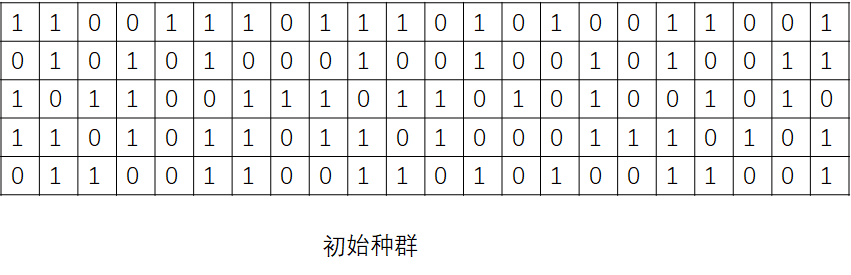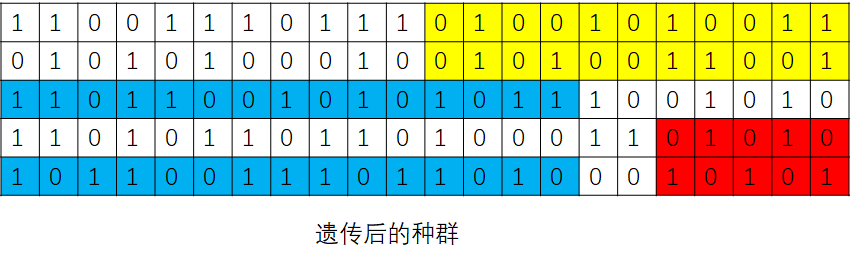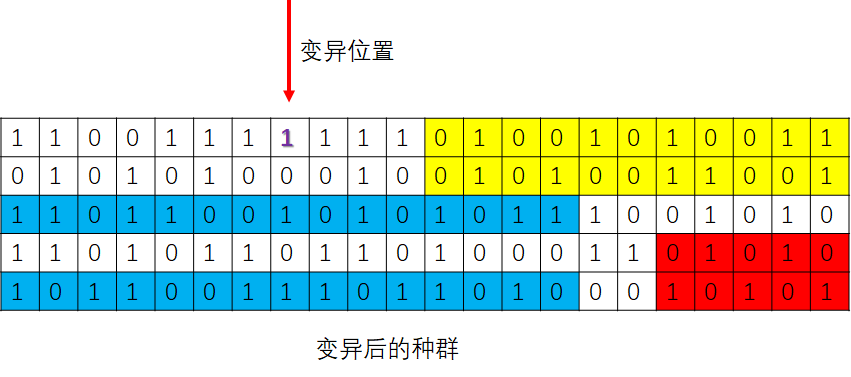这里需要指出的是，变异不仅仅发生在子代，变异会发生在整个种群的世世代代中，

上

一

代

的

变

异

会

伴

随

着

染

色

体

遗

传

给

下

一

代

.

\color{#00F}{上一代的变异会伴随着染色体遗传给下一代.}

4.多目标优化问题中的非支配排序
注意：这里所指的非支配排序只针对于多目标优化问题 什么是多目标？简单来说就是对于所要求解的问题来说，不仅仅只有一个目标那么简单，举个例子：在投资中，我们不仅仅期望得到一个收益较好的投资项目，同时也期望该风险较小，这便是一个双目标规划问题。 同样地，在路径规划问题中，如果我们需要得到一个路径及其过路费最小的方案，这也是一个双目标规划问题。 对于这种双目标或多目标问题该如何处理？ 现有的较为成熟的方法就是对各目标进行非支配排序。（这里仅仅介绍个人对于这种方法的一些见解，详细请大家参阅多目标遗传算法。 还是举路径这个例子。如果方案1的路径小于方案2并且过路费也比方案2少，那方案1不用说肯定优于方案2；如果方案1的路径小于方案3但是过路费比方案3高，那么方案1和方案2的优先顺序无法比较，则称方案1和方案3

非

支

配

\color{#00F}{非支配}

。这里每个方案都有多个与之非支配的方案，对所有方案找到非支配组合的过程也是非支配分层的过程，较快排序的算法为NSGA-II的快速非支配排序。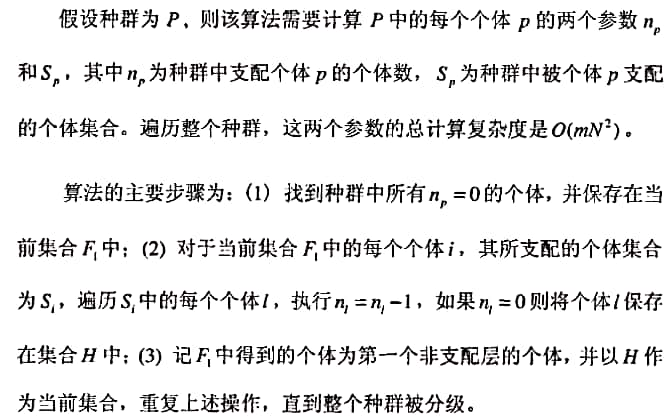整个种群被分层后，还是没法确定最优解，例如上述方案1、方案3、方案4，我们对这三种处于同一级别的方案进行筛选，其具体方法便是拥挤度（同层个体之间密度）比较。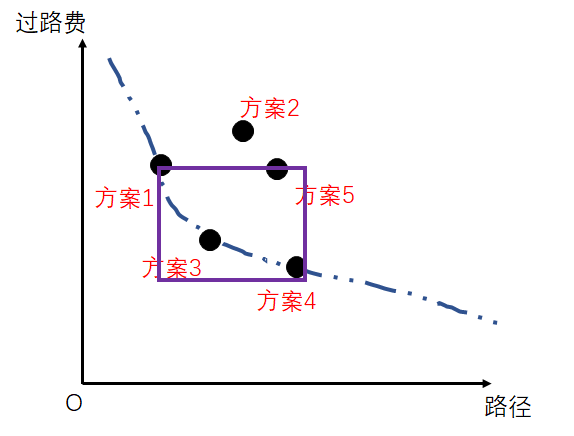定义拥挤度为图示矩形长，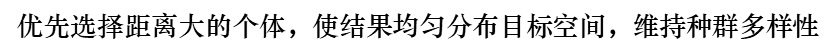二、路径问题的单目标遗传算法
对于路径问题时，由于路径为序列，我们一般采用采用十进制编码，用随机数列 作为染色体，其中 ；每一个随机序列都和种群中的一个个体相对应，例如一个 9 目标问题的一个染色体为 [0.23，0.82，0.45，0.74，0.87，0.11，0.56，0.69，0.78] 其中编码位置 代表目标 ，位置 的随机数表示目标 在巡回中的顺序，我们将这些随机数按升序排列得到如下巡回： 6－1－3－7－8－4－9－2－5
clear
M=50;%种群数量
G=1000;%最大的子代数
sj=[sj,sj(:,1)]';
n=size(sj,1);
d=zeros(n);
for i=1:n-1
for j=i+1:n
%         d(i,j)=6370*acos(cos(sj(i,1)-sj(j,1))*cos(sj(i,2))*cos(sj(j,2))+sin(sj(i,2))*sin(sj(j,2)));
d(i,j)=sqrt((sj(i,1)-sj(j,1))^2+(sj(i,2)-sj(j,2))^2);
end
end
d=d+d';%获得对称阵

path=linspace(1,n,n);
ZQ=zeros(M,n);%优秀种群初始化
YXGT=1;%优秀个体
while YXGT<=M
c=sort(2+floor((n-2)*rand(1,2)));
df=d(path(c(1)-1),path(c(2)))+d(path(c(1)),path(c(2)+1))-d(path(c(1)-1),path(c(1)))-d(path(c(2)),path(c(2)+1));
if df<0
path=[path(1:c(1)-1),path(sort(c(1):c(2),'descend')),path(c(2)+1:n)];
ZQ(YXGT,:)=path;
YXGT=YXGT+1;
end%采用2变化法得到优秀种群
end
mother=[ZQ(M-1,:);sort(rand(1,n))];father=[ZQ(M,:);sort(rand(1,n))];%选取优秀种群中的任意两个个体进行编码，获得父本和母本
long=zeros(4,1);
for p=1:G
t=floor(n*rand(1));
son=[mother(:,1:t),father(:,t+1:n)];
for i=2:n
for j=i:-1:2
if son(2,j)<son(2,j-1)
h=son(:,j-1);
son(:,j-1)=son(:,j);
son(:,j)=h;
end
end
end
daughter=[father(:,1:t),mother(:,t+1:n)];
for i=2:n
for j=i:-1:2
if daughter(2,j)<daughter(2,j-1)
h=daughter(:,j-1);
daughter(:,j-1)=daughter(:,j);
daughter(:,j)=h;
end
end
end
NEW=[father(1,:);mother(1,:);son(1,:);daughter(1,:)];

for j=1:n-1
long=long+d(NEW(1,j),NEW(1,j+1));
end
NEW=[NEW,long];
for l=2:4
for b=l:-1:2
if NEW(b,n+1)<NEW(b-1,n+1)
k=NEW(b-1,:);
NEW(b-1,:)=NEW(b,:);
NEW(b,:)=k;
end
end
end
mother=[NEW(1,1:n);sort(rand(1,n))];
father=[NEW(2,1:n);sort(rand(1,n))];
end
distance=0;
for i=1:n-1
distance=distance+d(path(i),path(i+1));
end
GreatX=sj(path,1);
GreatY=sj(path,2);
plot(GreatX,GreatY,'-*');
path
distance

展开全文线性代数
• 在静态路网模型的基础上构建时间依赖的动态路网模型数据库，进行动态路径规划问题研究。...研究结果表明，改进后的遗传算法具有更好的收敛效果和收敛稳定性，满足行进中的动态最优路径规划对求解精度和效率的要求。
• 该代码提出了遗传算法(GA)来优化3连杆(冗余)机器人的点对点轨迹规划 手臂。所提出的遗传算法的目标函数是在不超过最大值的情况下最小化旅行时间和空间 预先定义的扭矩，不与机器人工作空间中的任何障碍物发生碰撞。...
• 提出了一种基于遗传算法的时相关动态车辆路径规划模型。该模型将时变的交通信息和动态客户订单考虑在内，可以获得比较好的动态更新效率和优化结果，为此类动态车辆路径规划探索出了一条可行的途径。
• 考虑不确定性的遗传算法，将刘宝碇书的C语言代码转化为matlab代码。考虑不确定性的遗传算法，将刘宝碇书的C语言代码转化为matlab代码。
• 遗传算法学习——使用python做路径规划一、引言二、算法伪代码三、算法流程以及代码实现1、地图创建四、代码工程文档 一、引言   机器人控制过程中，路径规划是其中重要的一环。因此本文采用遗传算法对机器人移动...python 人工智能
• 遗传算法（GA）是无人艇路径规划系统中的一种有效方法，为了克服该算法易陷入局部最优早熟和收敛速度慢等缺陷，在不增加算法复杂度的前提下，基于数据驱动线性动态交叉策略提出了一种能够在最短时间内自适应动态调整...自适应
• 文中提出了基于神经网络和遗传算法动态环境下机器人动态避障路径规划方法，机器人工作空间动态环境信息的神经网络模型，并利用该模型建立机器人动态避障与神经网络输出的关系，然后将需规划路径的二维编码简化成一......